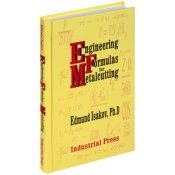•\$26.95

# Engineering Formulas for Metalcutting

## Overview

A unique and handy resource, Engineering Formulas for Metalcutting will enable users to calculate necessary speeds, feeds, and required machining power in order to maximize the productivity of cutting. Providing information on formulas and their applications in a concise and clearly arranged format, it describes mechanical properties of the most popular work materials, such as steels, cast irons, and nonferrous alloys. And it offers numerous formulas for calculating speeds, feeds, cutting forces, and machining power. What's more, practical examples of calculating the variety of such cutting parameters will make this a valuable source of knowledge in training and practice.

Features

• Linear regression equations for converting Rockwell, Vickers, Knoop, and Scleroscope hardness numbers into Brinell hardness numbers.
• Formulas and linear regression equations for calculating ultimate tensile strength of the most commonly used work materials in relationship with their hardness.
• Formulas for calculating the number of inserts simultaneously engaged with the workpiece depending on milling conditions.
• Formulas to calculate machining time when facing, cutoff, and deep grooving and for feed and radial forces in relationship with tangential force.
• Set of formulas to calculate overhang of boring bars made of tungsten heavy alloys and cemented carbides in comparison with a boring bar made of steel.
• Formulas for metal removal rate and for calculating tangential and axial forces.
• Establishes power constant values for most commonly used work materials.
Edmund Isakov
240 Pages, Hardcover
Published: June, 2005
ISBN: 9780831131746